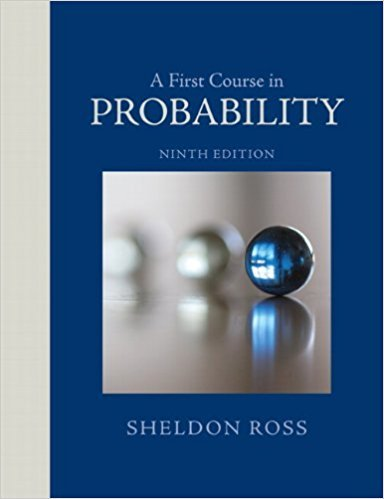×
Get Full Access to A First Course In Probability - 9 Edition - Chapter 3 - Problem 65p
Get Full Access to A First Course In Probability - 9 Edition - Chapter 3 - Problem 65p

×

# Assume, as in Example 3h, that 64 percent of twins are ofISBN: 9780321794772 63

## Solution for problem 65P Chapter 3

A First Course in Probability | 9th Edition

• Textbook Solutions
• 2901 Step-by-step solutions solved by professors and subject experts
• Get 24/7 help from StudySoup virtual teaching assistantsA First Course in Probability | 9th Edition

4 5 1 282 Reviews
20
5
Problem 65P

Problem 65P

Assume, as in Example 3h, that 64 percent of twins are of the same sex. Given that a newborn set of twins is of the same sex, what is the conditional probability that the twins arc identical?

Step-by-Step Solution:

Step 1 of 1

(a)

We are asked to find the conditional probability that the twins are identical given that a newborn set of twins is of the same sex.

Assume, as in Example 3h, that 64 percent of twins are of the same sex.

Letdenote the twins being identical.

The probability of twins being identical is given by using the example 3h,Letdenote the twins being of the same sex.

The probability of twins being of the same sex is given by,We are asked to find the conditional probabilityUsing Bayes’ rule we can write that[The conditional probability of twins being of the same sex given that the twins being identical would be, since the identical twins are always of the same sex]Hence the conditional probability that the twins are identical given that a newborn set of twins is of the same sex isStep 2 of 1

##### ISBN: 9780321794772

Unlock Textbook Solution